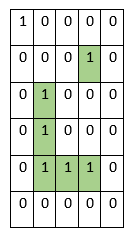# Program to count number of surrounded islands in the matrix in python

Suppose we have a binary matrix. Where 1 represents land and 0 represents water. As we know an island is a group of 1s that are grouped together whose perimeter is surrounded by water. We have to find the number of completely surrounded islands.

So, if the input is likethen the output will be 2, as there are three islands, but two of them are completely surrounded.

To solve this, we will follow these steps −

• Define a function dfs() . This will take i, j
• if i and j are not in range of matrix, then
• return False
• if matrix[i, j] is same as 0, then
• return True
• matrix[i, j] := 0
• a := dfs(i + 1, j)
• b := dfs(i - 1, j)
• c := dfs(i, j + 1)
• d := dfs(i, j - 1)
• return a and b and c and d
• From the main method do the following:
• R := row count of matrix, C := column count of matrix
• ans := 0
• for i in range 0 to R, do
• for j in range 0 to C, do
• if matrix[i, j] is same as 1, then
• if dfs(i, j) is true, then
• ans := ans + 1
• return ans

Let us see the following implementation to get better understanding −

## Example

Live Demo

class Solution:
def solve(self, matrix):
def dfs(i, j):
if i < 0 or j < 0 or i >= R or j >= C:
return False
if matrix[i][j] == 0:
return True
matrix[i][j] = 0
a = dfs(i + 1, j)
b = dfs(i - 1, j)
c = dfs(i, j + 1)
d = dfs(i, j - 1)
return a and b and c and d

R, C = len(matrix), len(matrix)
ans = 0
for i in range(R):
for j in range(C):
if matrix[i][j] == 1:
if dfs(i, j):
ans += 1
return ans

ob = Solution()
matrix = [
[1, 0, 0, 0, 0],
[0, 0, 0, 1, 0],
[0, 1, 0, 0, 0],
[0, 1, 0, 0, 0],
[0, 1, 1, 1, 0],
[0, 0, 0, 0, 0]
]
print(ob.solve(matrix))

## Input

matrix = [
[1, 0, 0, 0, 0],
[0, 0, 0, 1, 0],
[0, 1, 0, 0, 0],
[0, 1, 0, 0, 0],
[0, 1, 1, 1, 0],
[0, 0, 0, 0, 0] ]

## Output

2